# python可视化：matplotlib学习笔记

matplotlib是一个桌面绘图包，用于绘制(主要是二维的)发表用的图。该项目由John Hunter在2002年启动，目的是在Python中使用类似matlab的绘图界面。matplotlib和IPython协作简化了IPython shell(现在是Jupyter笔记本)中的交互式绘图。matplotlib支持所有操作系统上的各种GUI后端，此外还可以导出可视化到所有常见的格式中(PDF、SVG、JPG、PNG、BMP、GIF等)。

%matplotlib notebook

In : import matplotlib.pyplot as plt

In : import numpy as np

In : data = np.arange(10)

In : data
Out: array([0, 1, 2, 3, 4, 5, 6, 7, 8, 9])

In : plt.plot(data)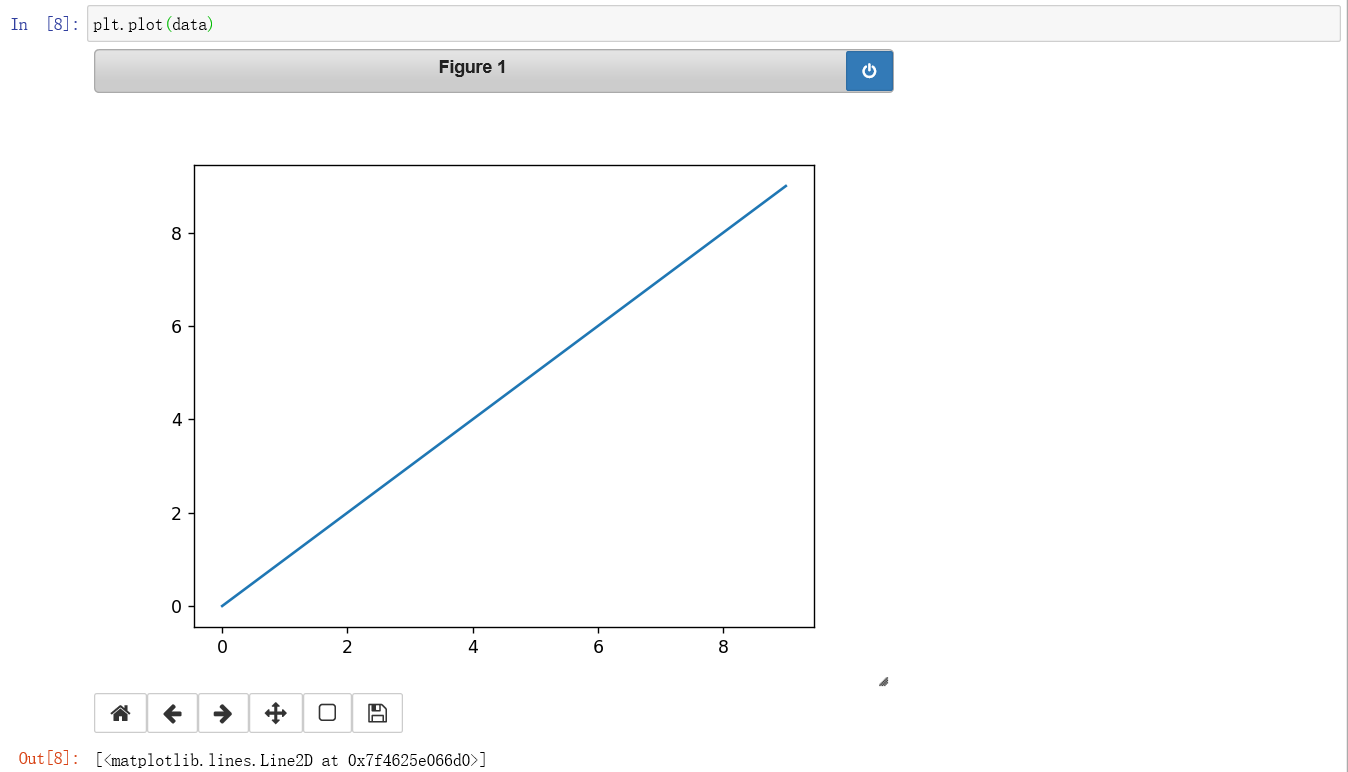（一）Figures and Subplots

In : fig = plt.figure()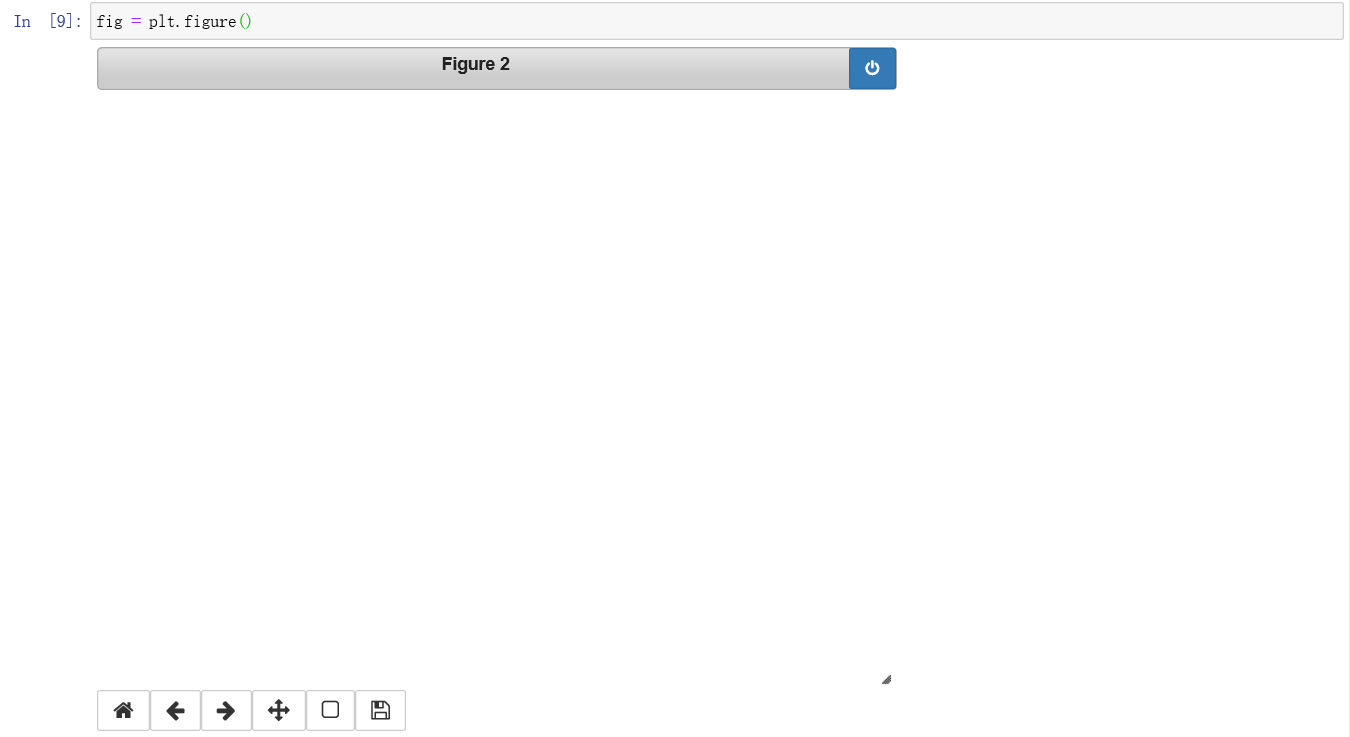In : ax1 = fig.add_subplot(2, 2, 1)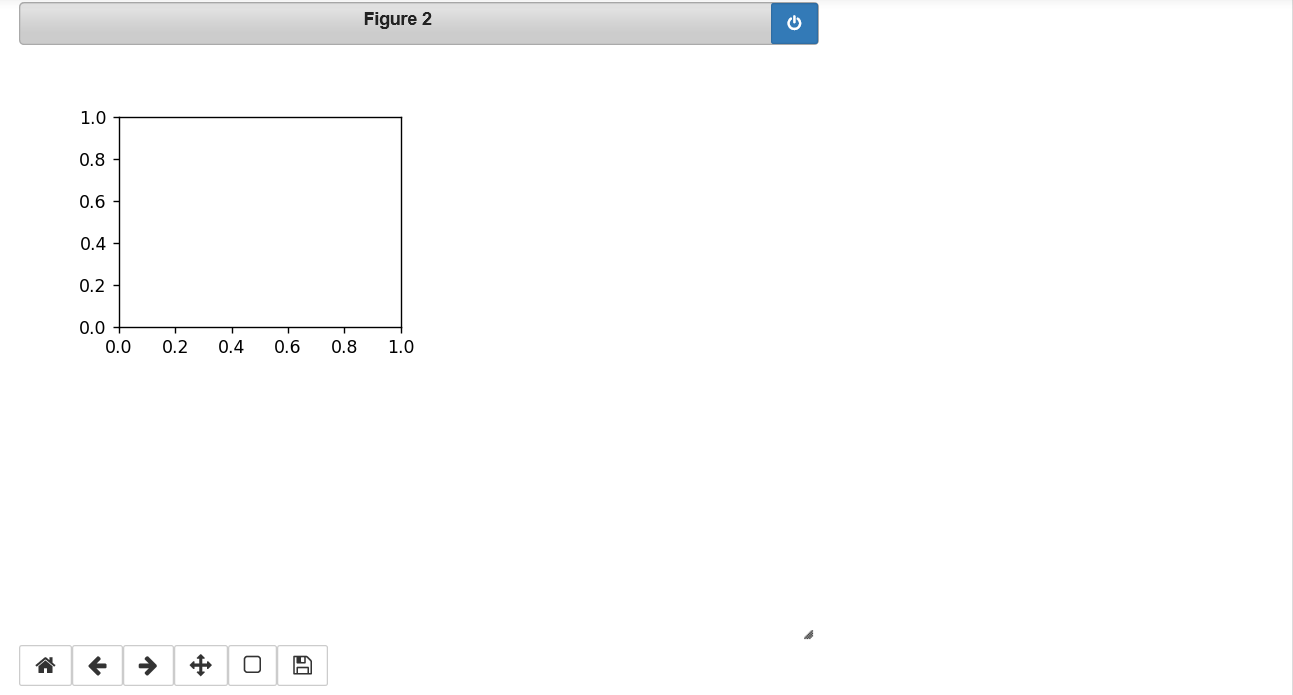In : ax2 = fig.add_subplot(2, 2, 2)
ax3 = fig.add_subplot(2, 2, 3)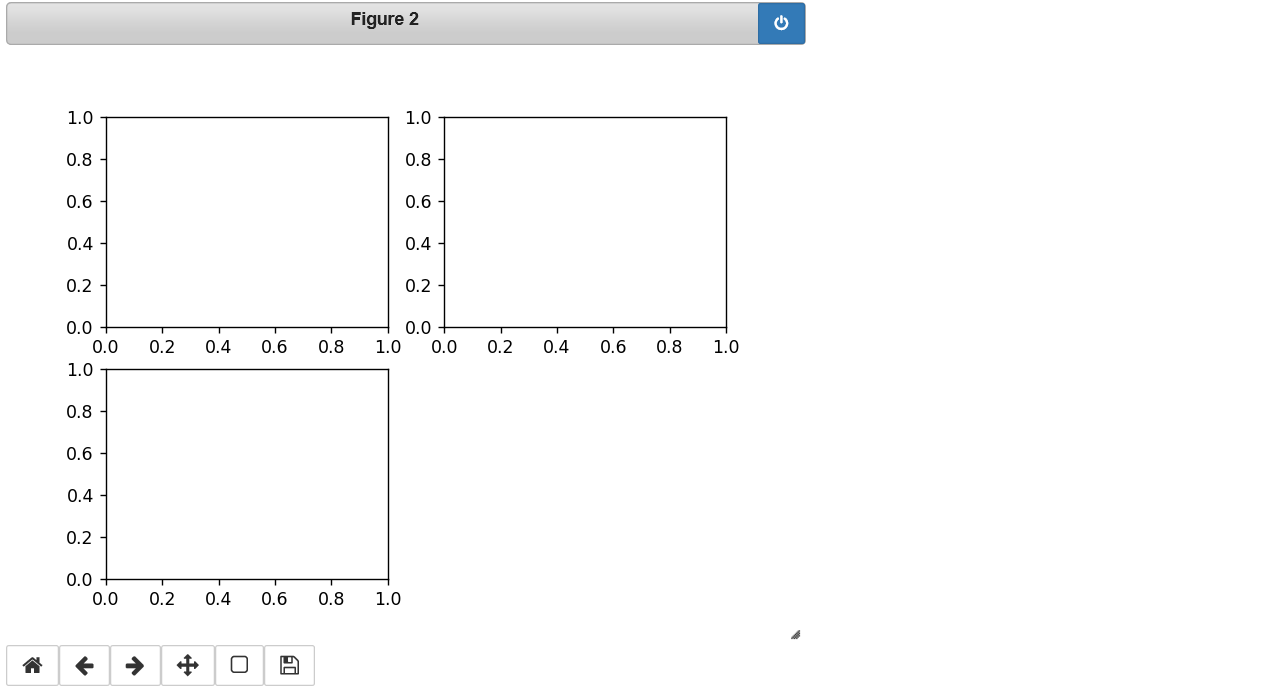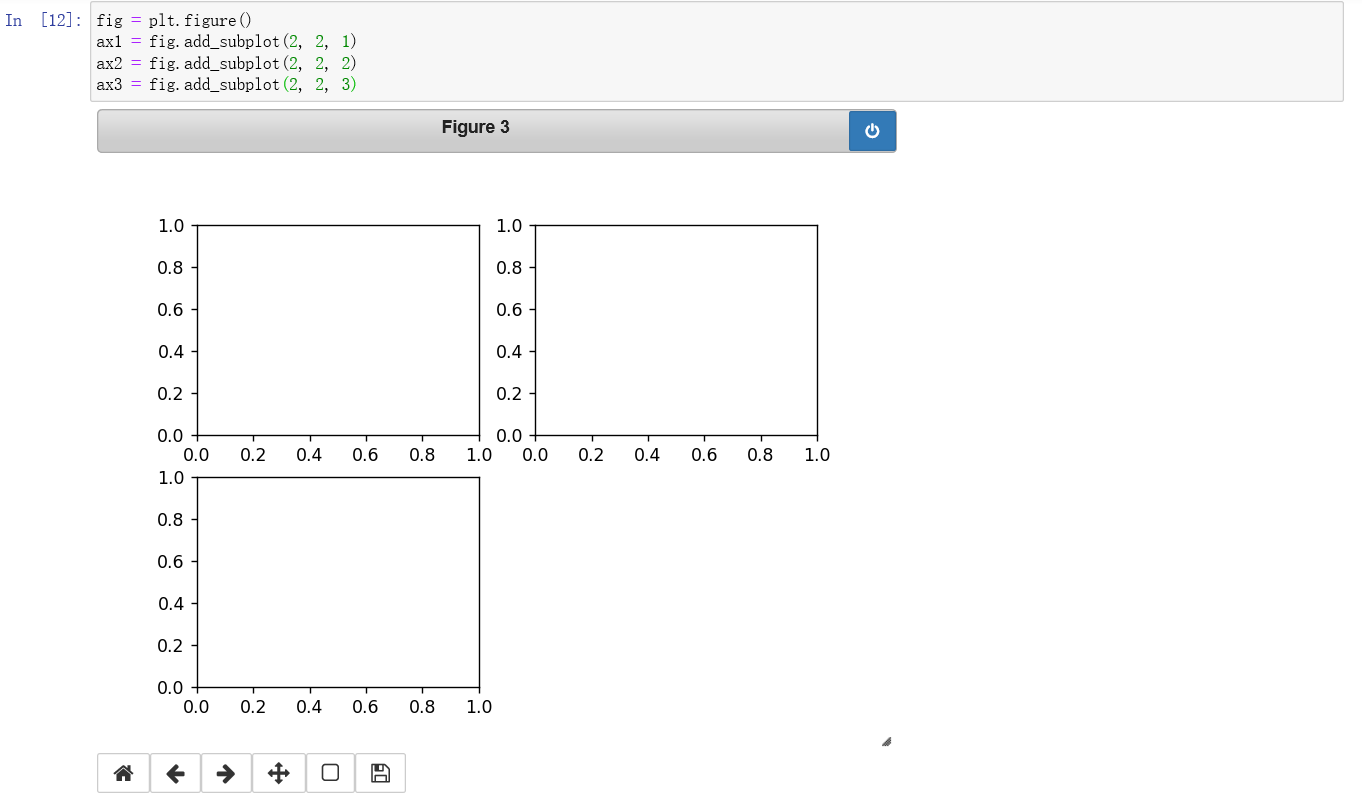In : plt.plot([1.5, 3.5, -2, 1.6])
Out : [<matplotlib.lines.Line2D at 0x7f462574f590>]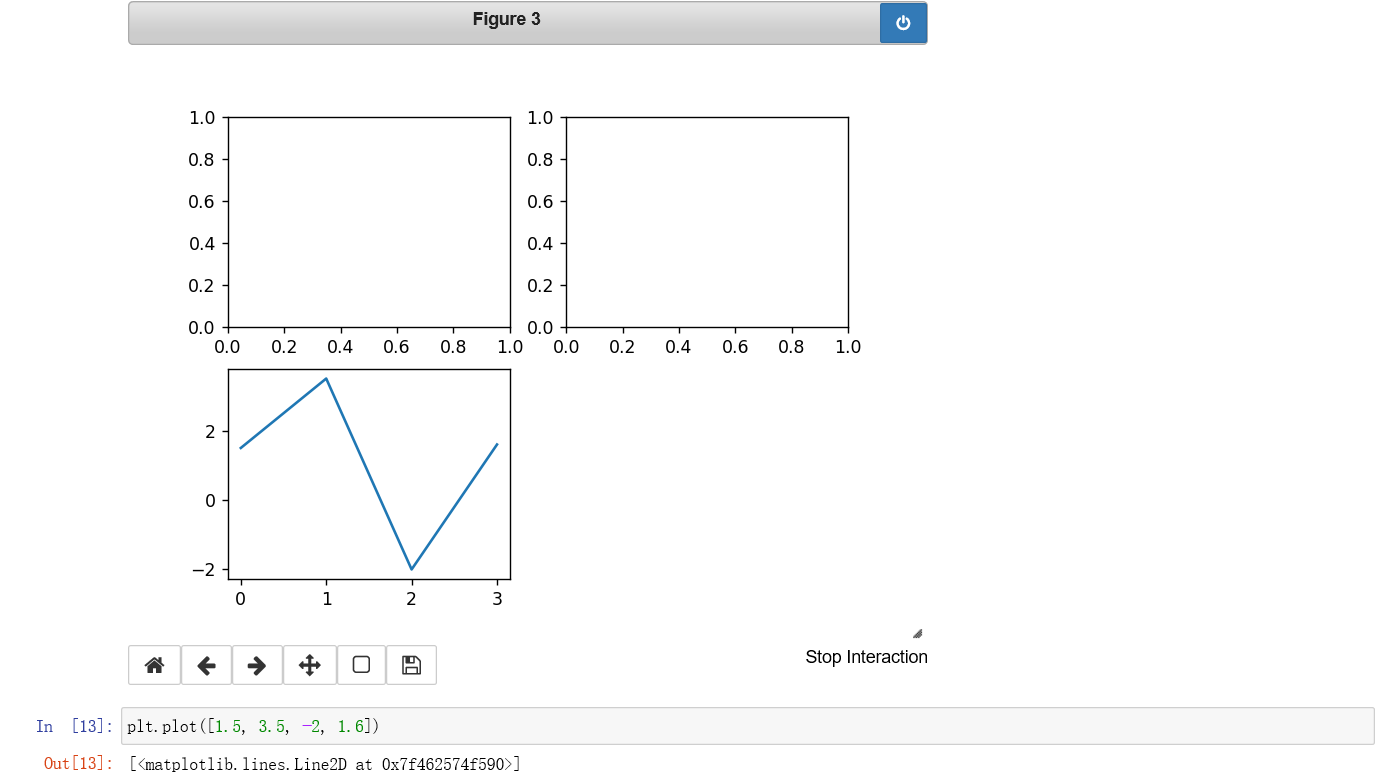In : plt.plot(np.random.randn(50).cumsum(), 'k--')
Out : [<matplotlib.lines.Line2D at 0x7f4625737110>]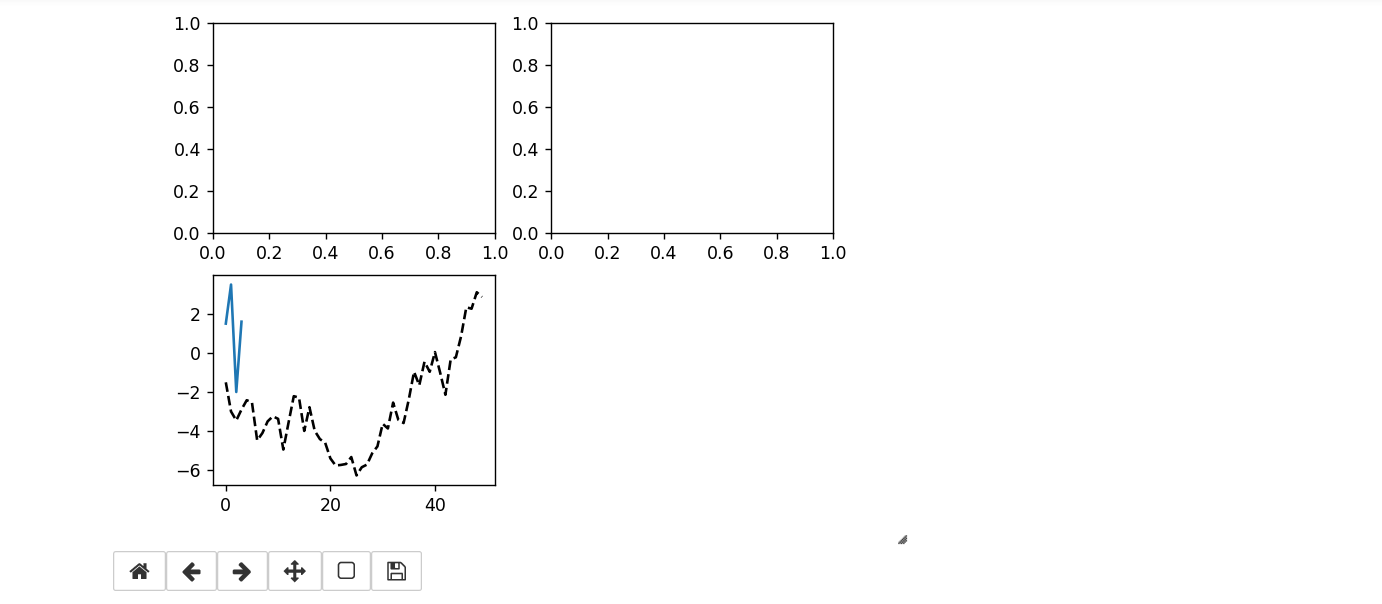'k--'的意思是在matplotlib里画一条黑色的虚线。在其他空白的地方添加图形：

In : _ = ax1.hist(np.random.randn(100), bins=20, color='k', alpha=0.3)
ax2.scatter(np.arange(30), np.arange(30) + 3 * np.random.randn(30))
Out : <matplotlib.collections.PathCollection at 0x7f46256f75d0>

（1）Adjusting the spacing around subplots调整子图之间的间距

subplots_adjust(left=None, bottom=None, right=None, top=None, wspace=None, hspace=None)

wspace和hspace控制图形宽度和高度的百分比，以控制子图之间的空间。下面是一个小例子：

In : fig, axes = plt.subplots(2, 2, sharex=True, sharey=True)
for i in range(2):
for j in range(2):
axes[i, j].hist(np.random.randn(500), bins=50, color='k', alpha=0.5)
plt.subplots_adjust(wspace=0, hspace=0)#这里设置空隙是0，子图之间就是连接起来的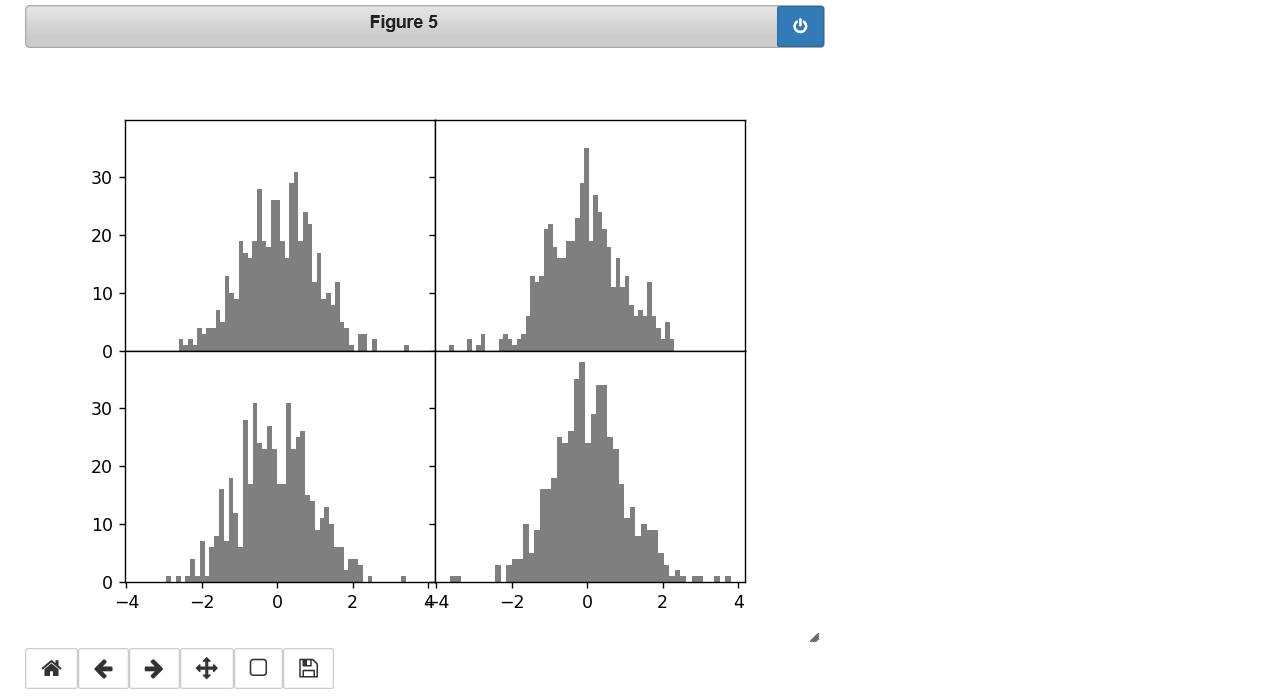（二）Colors, Markers, and Line Styles
Matplotlib的主函数接受x和y坐标数组，也可以接受表示颜色和线条样式的字符串缩写。例如，要用绿色破折号绘制x和y，用如下代码:

ax.plot(x, y, 'g--')

ax.plot(x, y, linestyle='--', color='g')

In : fig = plt.figure()
from numpy.random import randn
plt.plot(randn(30).cumsum(), 'ko--')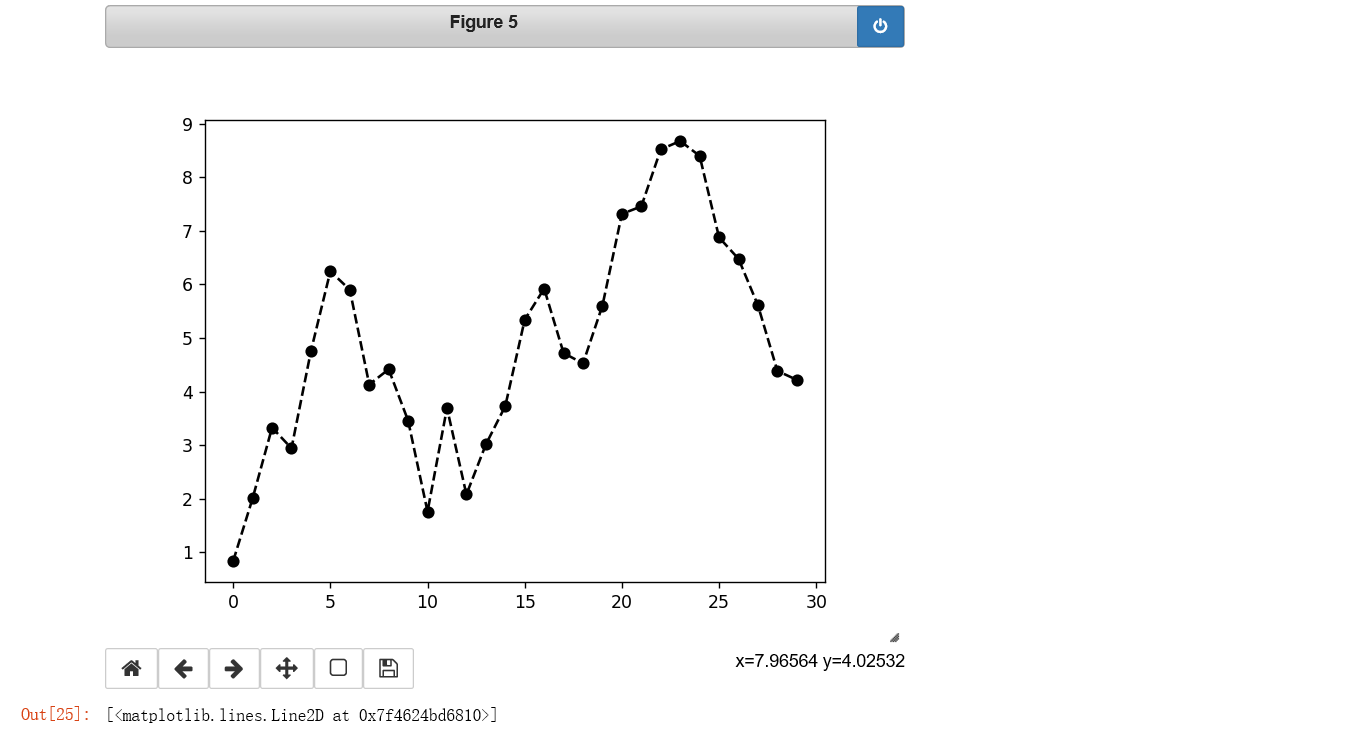（三）Ticks, Labels, and Legends

•不设置参数返回当前参数值(例如：plt.xlim())返回当前的x轴绘制范围)
•调用参数设置参数值(例如：plt.xlim([0, 10])，x轴范围为0到10)
（1）设置标题，坐标轴标签，刻度，刻度标签

In : fig = plt.figure() #创建一个新的空白图
ax = fig.add_subplot(1, 1, 1) #设置图的个数，1行*1列，将下面的代码绘制到第一个图里
ax.plot(np.random.randn(1000).cumsum()) #绘图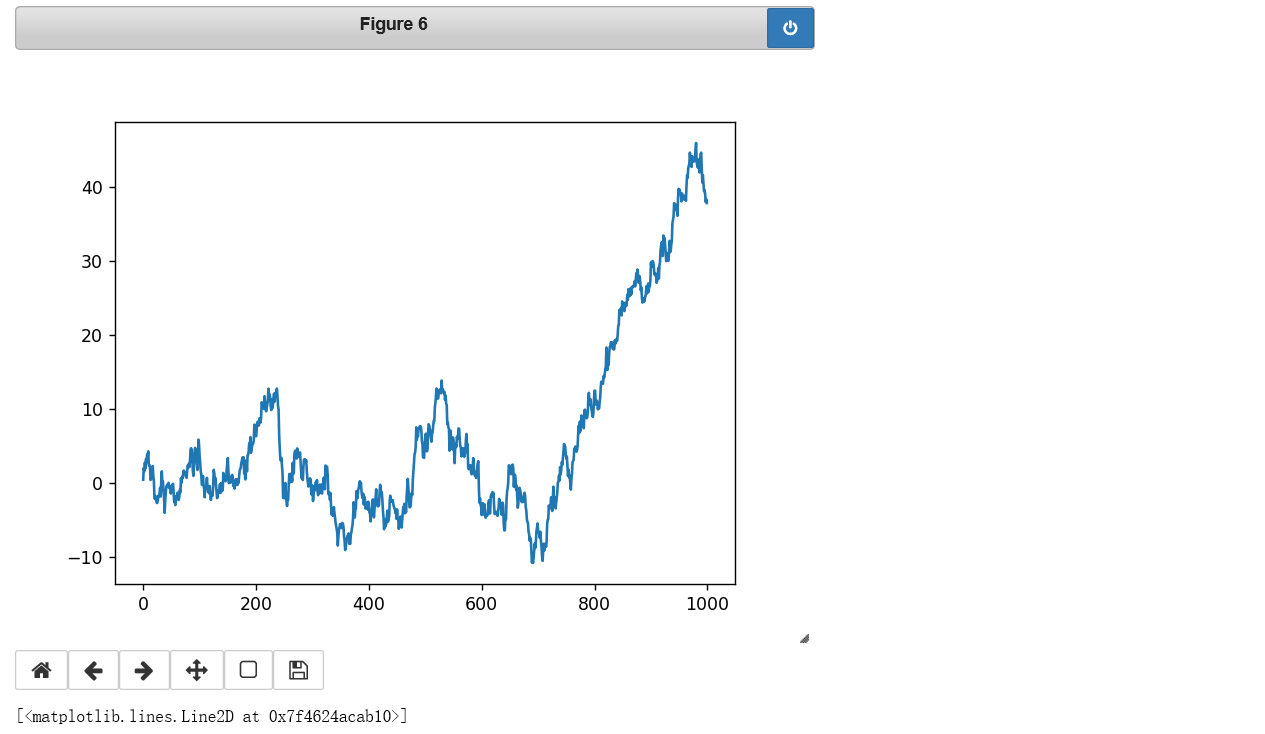In : ticks = ax.set_xticks([0, 250, 500, 750, 1000])
labels = ax.set_xticklabels(['one', 'two', 'three', 'four', 'five'],rotation=30, fontsize='small')
ax.set_xlabel('Stages')
ax.set_title('My first matplotlib plot')

In : props = {
'title': 'My first matplotlib plot',
'xlabel': 'Stages'
}
ax.set(**props)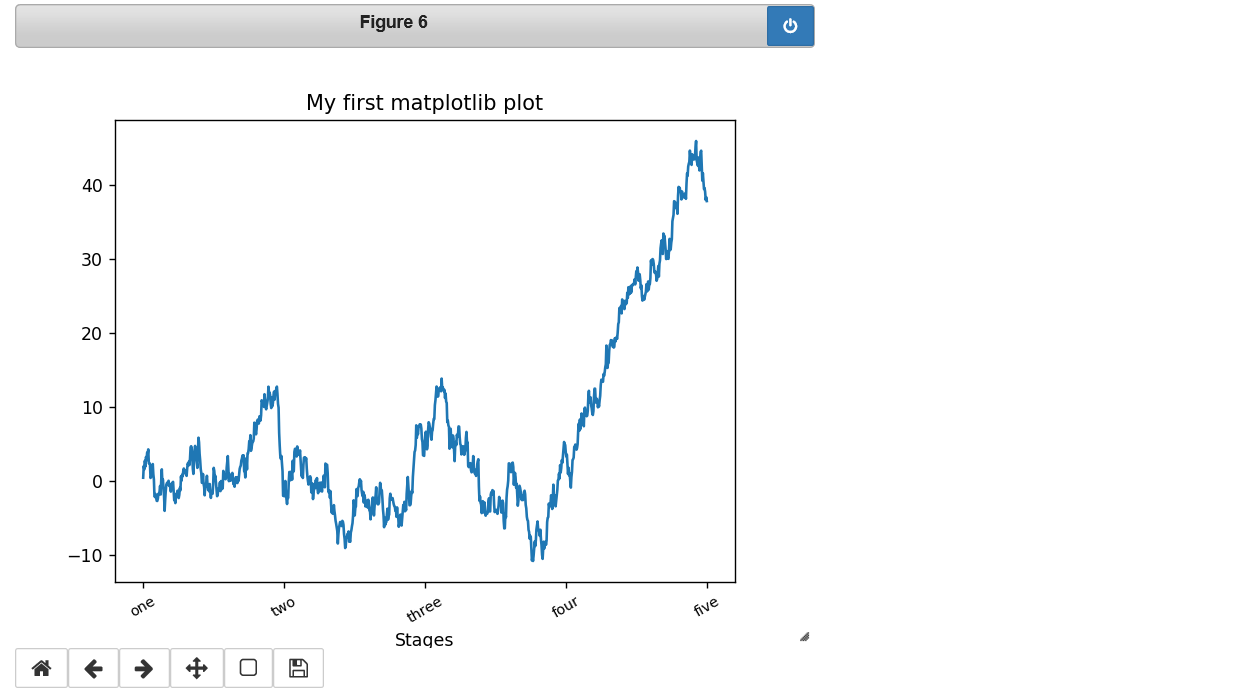（2）添加注释（Legends）

In : from numpy.random import randn
fig = plt.figure()
ax = fig.add_subplot(1, 1, 1)
ax.plot(randn(1000).cumsum(), 'k', label='one') #实线
ax.plot(randn(1000).cumsum(), 'k--', label='two') #虚线
ax.plot(randn(1000).cumsum(), 'k.', label='three') #点图
ax.legend(loc='best') #loc='best'指选择一个最合适的位置来绘图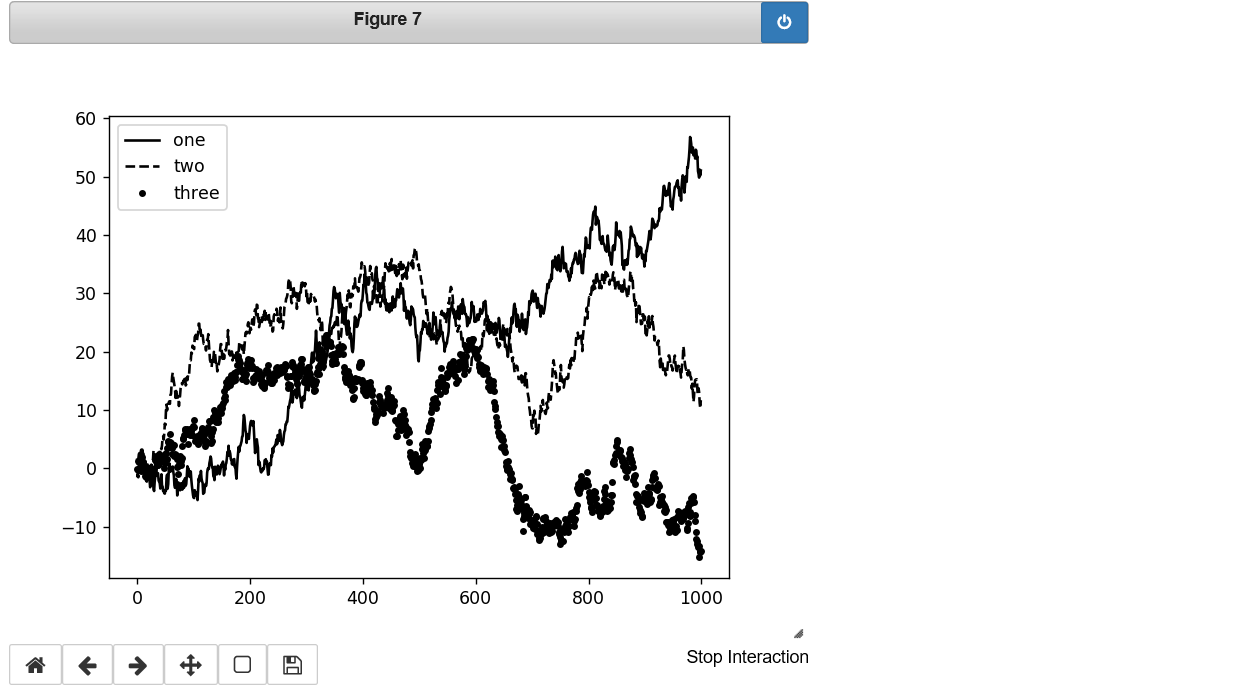（四）Annotations and Drawing on a Subplot

ax.text(x, y, 'Hello world!', family='monospace', fontsize=10)

In : import pandas as pd

In : data = pd.read_csv('./spx.csv', index_col=0, parse_dates=True)

In : data
Out:
AAPL   MSFT    XOM      SPX
2003-01-02    7.40  21.11  29.22   909.03
2003-01-03    7.45  21.14  29.24   908.59
2003-01-06    7.45  21.52  29.96   929.01
2003-01-07    7.43  21.93  28.95   922.93
2003-01-08    7.28  21.31  28.83   909.93
...            ...    ...    ...      ...
2011-10-10  388.81  26.94  76.28  1194.89
2011-10-11  400.29  27.00  76.27  1195.54
2011-10-12  402.19  26.96  77.16  1207.25
2011-10-13  408.43  27.18  76.37  1203.66
2011-10-14  422.00  27.27  78.11  1224.58

[2214 rows x 4 columns]

In : from datetime import datetime
import pandas as pd

fig = plt.figure() #创建新图形
ax = fig.add_subplot(1, 1, 1) #只创建一个图形

spx = data['SPX'] #把最后一列赋给一个变量

spx.plot(ax=ax, style='k-')
crisis_data = [
(datetime(2007, 10, 11), 'Peak of bull market'),
(datetime(2008, 3, 12), 'Bear Stearns Fails'),
(datetime(2008, 9, 15), 'Lehman Bankruptcy')
]  #对三个特定点进行标注

for date, label in crisis_data:
ax.annotate(label, xy=(date, spx.asof(date) + 75),
xytext=(date, spx.asof(date) + 225),
arrowprops=dict(facecolor='black', headwidth= 4, width=2,headlength=4),
horizontalalignment='left',verticalalignment='top')
# Zoom in on 2007-2010
ax.set_xlim(['1/1/2007', '1/1/2011']) #限制X轴的范围
ax.set_ylim([600, 1800]) #限制Y轴范围
ax.set_title('Important dates in the 2008-2009 financial crisis') #图标题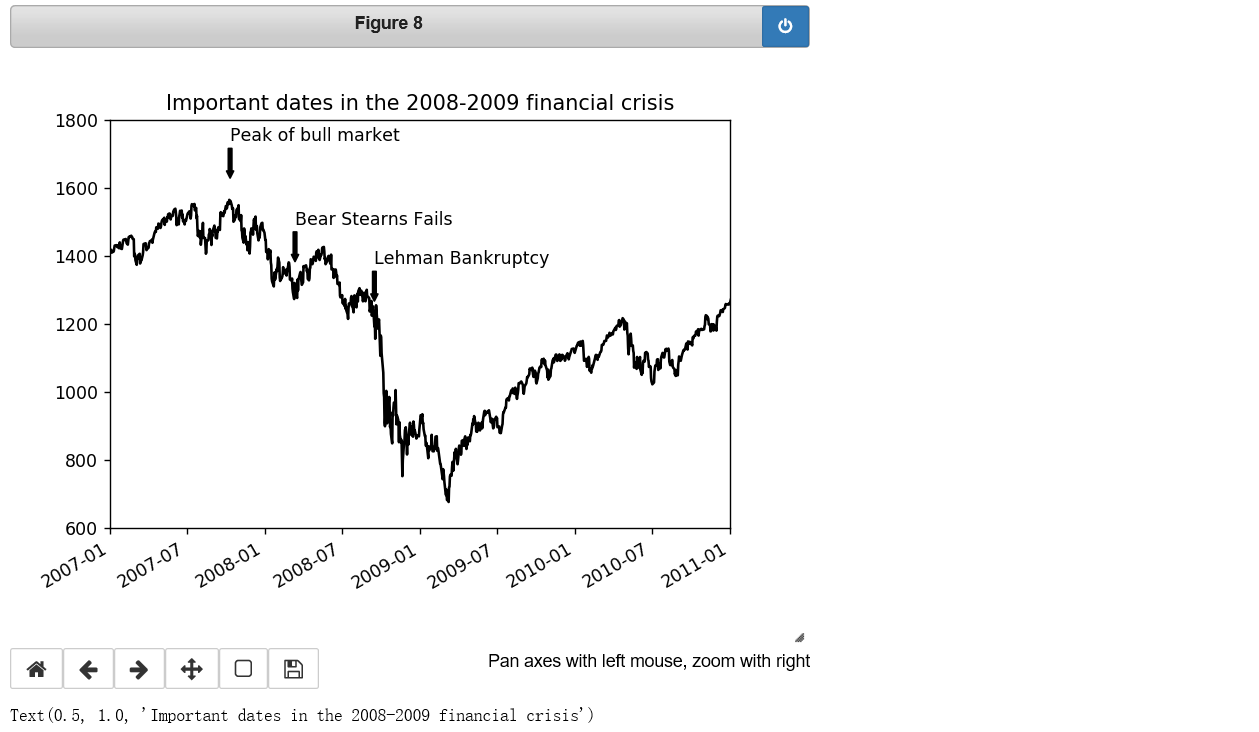In : fig = plt.figure()
ax = fig.add_subplot(1, 1, 1)

rect = plt.Rectangle((0.2, 0.75), 0.4, 0.15, color='k', alpha=0.3) #(0.2,0.75)是四边形左下角的坐标位置，0.4代表长度，0.15代表高度
circ = plt.Circle((0.7, 0.2), 0.15, color='b', alpha=0.3) #(0.7,0.2)是椭圆形中心点的位置，0.15代表半径
pgon = plt.Polygon([[0.15, 0.15], [0.35, 0.4], [0.2, 0.6]],color='g', alpha=0.5) #三个[]里代表三角形三个点的坐标位置

ax.add_patch(rect)
ax.add_patch(circ)
ax.add_patch(pgon)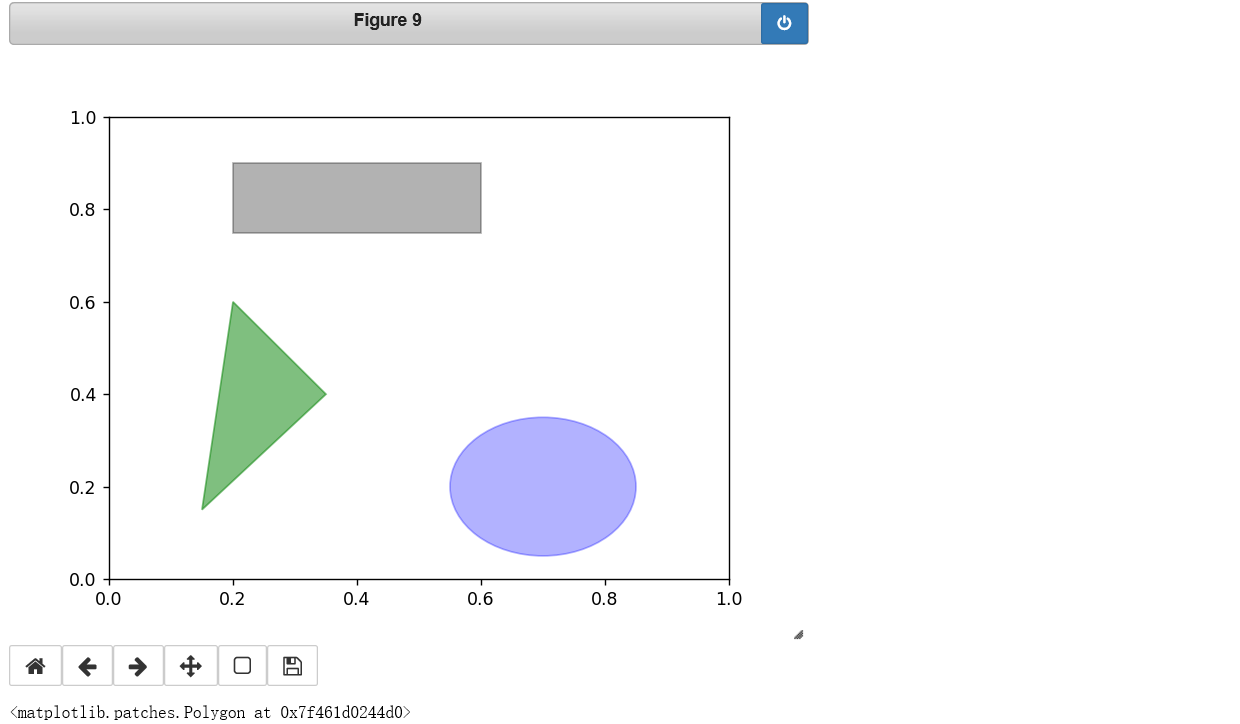（五）Saving Plots to File保存

plt.savefig('figpath.svg')

plt.savefig('figpath.png', dpi=400, bbox_inches='tight')

（六）matplotlib Configuration配置
matplotlib配置了配色方案和默认设置，主要用于准备发布的图形。有一点很方便的是，几乎所有的默认参数都可以通过一组全局参数来设置，这些参数包括图形大小、子图间距、颜色、字体大小、网格样式等等。Python里的rc方法可以进行全局的参数设置。例如，要设置全局默认图形大小为10×10，可以输入:

plt.rc('figure', figsize=(10, 10))

font_options = {'family' : 'monospace',
'weight' : 'bold',
'size' : 'small'}
plt.rc('font', **font_options)

20人点赞
Python学习笔记07-18397
06-301430
03-01327
03-292191
07-23988
03-288264
07-14222
08-16817
03-288884
12-225328
©️2020 CSDN 皮肤主题: 大白 设计师:CSDN官方博客